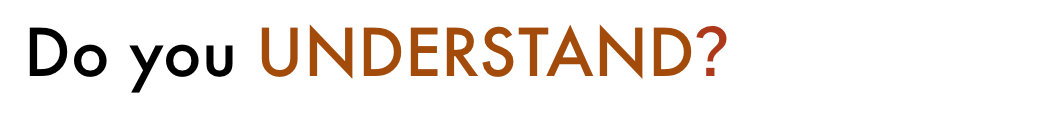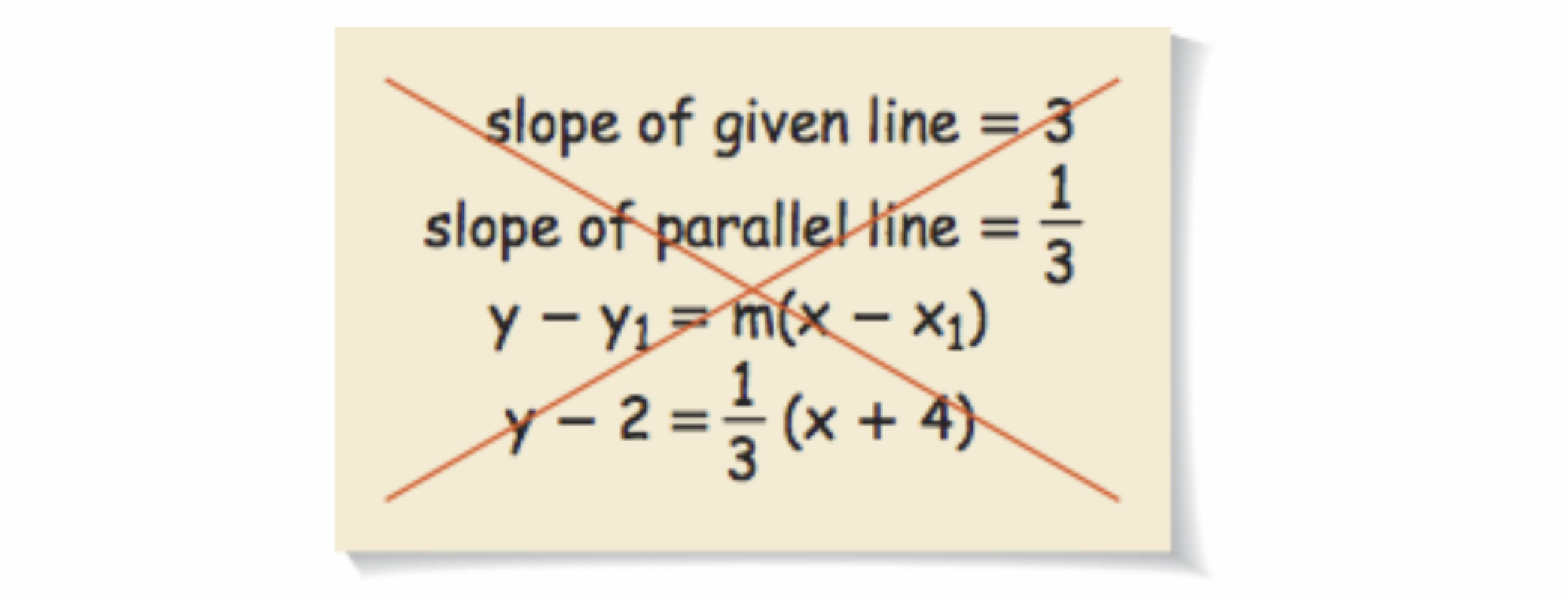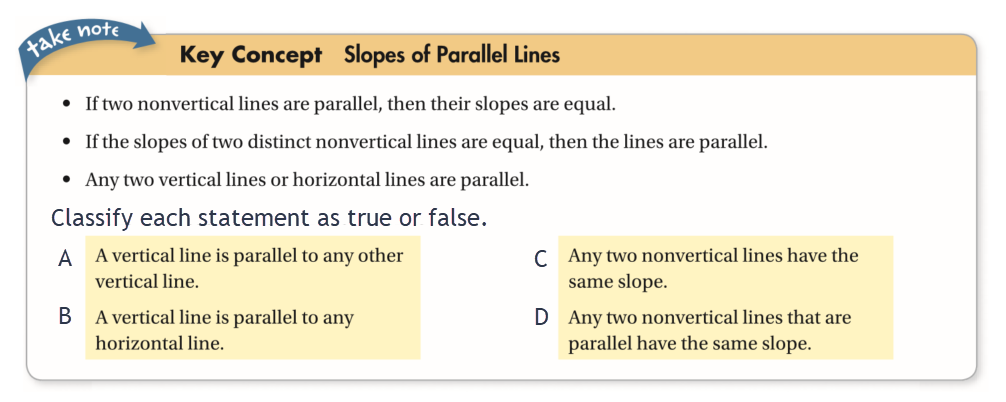Geometry 3-8 Slopes of Parallel and Perpendicular Lines
starstarstarstarstarstarstarstarstarstar
by Matthew Richardson
| 20 Questions
Note from the author:
A complete formative lesson with embedded slideshow, mini lecture screencasts, checks for understanding, practice items, mixed review, and reflection. I create these assignments to supplement each lesson of Pearson's Common Core Edition Algebra 1, Algebra 2, and Geometry courses. See also mathquest.net and twitter.com/mathquestEDU.
The outlined content above was added from outside of Formative.
The outlined content above was added from outside of Formative.1
2
1
10 pts
Solve It! You and a friend enjoy exercising together. One day, you are about to go running when your friend receives a phone call. You decide to start running and tell your friend to catch up after the call. The red line represents you and the blue line represents your friend. Will your friend catch up?
Yes
No
The outlined content above was added from outside of Formative.3
3
10 pts
Problem 1 Got It?
A
B
C
D
The outlined content above was added from outside of Formative.4
4
10 pts
Problem 2 Got It?
A
B
C
D
The outlined content above was added from outside of Formative.5
5
10 pts
Problem 3 Got It? If a given line has a slope of 1/2, what is the slope of a perpendicular line?
-1/2
2
-2
Undefined6
6
10 pts
Problem 3 Got It?
A
B
C
D
The outlined content above was added from outside of Formative.7
7
10 pts
Problem 4 Got It?
A
B
C
D
The outlined content above was added from outside of Formative.8
8
10 pts
Problem 5 Got It? What is an equation of the line representing the player's path?

A
B
CFor each set of points below, line AB contains points A and B. Line CD contains points C and D. Are Line AB and line CD parallel, perpendicular, or neither?
9
10 pts
A(-8, 3), B(-4, 11), C(-1, 3), D(1, 2)

Lines AB and CD are __________.
parallel
perpendicular
neither parallel nor perpendicular
10
10 pts
A(3, 5), B(2, -1), C(7, -2), D(10, 16)

Lines AB and CD are __________.
parallel
perpendicular
neither parallel nor perpendicular
11
10 pts
A(3, 1), B(4, 1), C(5, 9), D(2, 6)

Lines AB and CD are __________.
parallel
perpendicular
neither parallel nor perpendicular
12
10 pts
What is an equation of the line perpendicular to the line y = -4x + 1 that contains (2, -3)?

A
B
C
D13
13
10 pts
Error Analysis: Your classmate tries to find an equation for a line parallel to y = 3x - 5 that contains (-4, 2). What is your classmate's error?
14
10 pts
Compare and Contrast: What are the differences between the equations of parallel lines and the equations of perpendicular lines?15
10 pts
Review Lesson 3-7: Write an equation for the line containing the points.

y = -(1/2)x + 3
y = -3x + 2
y = 2x + (1/2)
y = -x + 616
16
10 pts
Review Lessons 1-5 & 2-6:
Are ∠1 and ∠2 congruent?
• Diagram A
• Diagram B
• Congruent
• Not contruent17
17
10 pts
Vocabulary Review: Classify the relationship between the lines.
• a and b
• b and the x-axis
• a and the y-axis
• a and the x-axis
• Parallel
• Perpendicular
18
50 pts
Vocabulary Review: Conisder the conditional statement below and categorize each of the items.

Conditional: If a polygon is a triangle, then the sum of the measures of its angles is 180.
• the sum of the measures of its angles is 180
• If the sum of the measures of the angles of a polygon is 180, then the polygon is a triangle.
• If the sum of the measures of the angles of a polygon is not 180, then the polygon is not a triangle.
• a polygon is a triangle
• If a polygon is not a triangle, then the sum of the measures of its angles is not 180.
• Hypothesis
• Conclusion
• Converse
• Inverse
• Contrapositive19
19
10 pts
Slopes of Parallel Lines: Classify each statement.
• A
• B
• C
• D
• True
• False
20
10 pts
Reflect: Math Success
Add to my formatives list

Formative uses cookies to allow us to better understand how the site is used. By continuing to use this site, you consent to the Terms of Service and Privacy Policy.